Next: Spatial Up: Discrete Labels Mixture Model Previous: Non-spatial without Class Proportions

### Non-spatial with Class Proportions

Again, we assume spatial independence between the classification labels as in equation 2. However, taking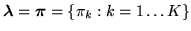, where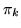are the adaptive global class proportion parameters, the prior on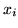is now: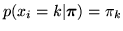(4)

The global class proportions,, are the relative weighting of each of the distributions in the mixture. The prior on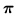is non-informative (uniform) over the range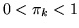, and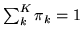. Using this in equation 1, the posterior becomes: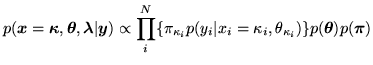(5)